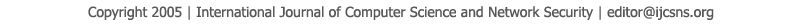To search, Click below search items.All Published Papers Search Service
 Title Author Year Keyword
 Title Computational and Analytical solution of Fractional order Linear Partial Differential equations using Sumudu Transform and its properties Author Faryal Aijaz Ansari, Israr Ahmed, Kashif Ali Dharejo, Abdul Naeem Kalhoro Citation Vol. 18  No. 9  pp. 43-50 Abstract In this work, an analytical outcome of fractional order Linear Partial differential equations is solved, which is collective result of the Sumudu Transform and its differential and integral properties and shows its ability on fractional order Linear Partial differential equations. In this method the outcomes are achieved in the form of quickly convergent infinite series with simply computable terms and approximate solutions of fractional orders are compared graphically. It is mention that this technique removes linearization and biologically unrealistic assumptions and gives an effective solution. The achieved outcomes are calculated using the symbolic calculus software Maple 16. This technique was undoubtedly very effective and powerful scheme in finding the solutions of approximate and numerical solutions as well as exact solutions. Keywords Time Fractional Diffusion equation, One-dimensional Fractional wave-like equation, Two dimensional Fractional heat-like equation, Fractional Sumudu Transform method, Fractional integrals and derivatives for Sumudu Transform. URL http://paper.ijcsns.org/07_book/201809/20180905.pdf